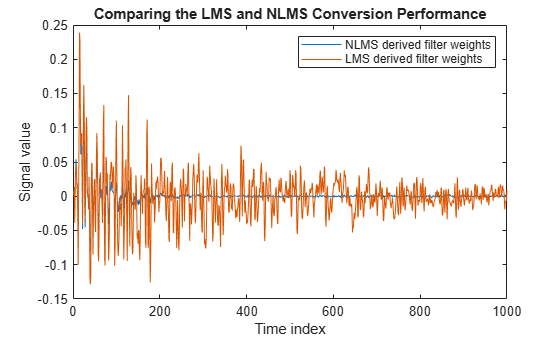# Compare Convergence Performance Between LMS Algorithm and Normalized LMS Algorithm

An adaptive filter adapts its filter coefficients to match the coefficients of an unknown system. The objective is to minimize the error signal between the output of the unknown system and the output of the adaptive filter. When these two outputs converge and match closely for the same input, the coefficients are said to match closely. The adaptive filter at this state resembles the unknown system. This example compares the rate at which this convergence happens for the normalized LMS (NLMS) algorithm and the LMS algorithm with no normalization.

### Unknown System

Create a `dsp.FIRFilter` that represents the unknown system. Pass the signal `x` as an input to the unknown system. The desired signal `d` is the sum of the output of the unknown system (FIR filter) and an additive noise signal `n`.

```filt = dsp.FIRFilter; filt.Numerator = fircband(12,[0 0.4 0.5 1],[1 1 0 0],[1 0.2],... {'w' 'c'}); x = 0.1*randn(1000,1); n = 0.001*randn(1000,1); d = filt(x) + n;```

Create two `dsp.LMSFilter` objects, with one set to the LMS algorithm, and the other set to the normalized LMS algorithm. Choose an adaptation step size of 0.2 and set the length of the adaptive filter to 13 taps.

```mu = 0.2; lms_nonnormalized = dsp.LMSFilter(13,'StepSize',mu,... 'Method','LMS'); lms_normalized = dsp.LMSFilter(13,'StepSize',mu,... 'Method','Normalized LMS');```

Pass the primary input signal `x` and the desired signal `d` to both the variations of the LMS algorithm. The variables `e1 `and `e2` represent the error between the desired signal and the output of the normalized and nonnormalized filters, respecitvely.

```[~,e1,~] = lms_normalized(x,d); [~,e2,~] = lms_nonnormalized(x,d);```

Plot the error signals for both variations. The error signal for the NLMS variant converges to zero much faster than the error signal for the LMS variant. The normalized version adapts in far fewer iterations to a result almost as good as the nonnormalized version.

```plot([e1,e2]); title('Comparing the LMS and NLMS Conversion Performance'); legend('NLMS derived filter weights', ... 'LMS derived filter weights','Location', 'NorthEast'); xlabel('Time index') ylabel('Signal value')```## Related Topics

 Hayes, Monson H., Statistical Digital Signal Processing and Modeling. Hoboken, NJ: John Wiley & Sons, 1996, pp.493–552.

 Haykin, Simon, Adaptive Filter Theory. Upper Saddle River, NJ: Prentice-Hall, Inc., 1996.

Watch now